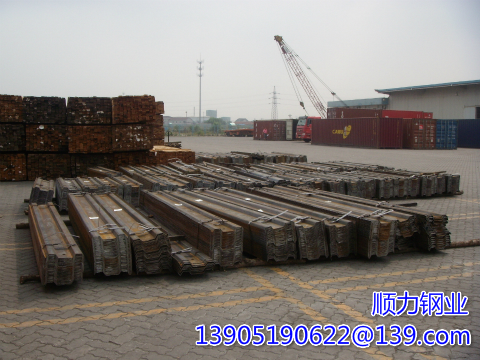» » » Function of Design Safety Factor for Retaining Wall of sheet piling

# Function of Design Safety Factor for Retaining Wall of sheet piling

Views:90

There are many ways to calculate the embedment depth of sheet piling, and these methods are usually based on experience, and soil at the point of failure to reach the ultimate master / passive state. According to the limit state of the earth pressure map, However, a limit state means that the wall will inevitably fail, at this time, we need to introduce a safety factor to control the earth pressure at a reasonable value, to avoid wall failure .The safety factor can have a different effect:
1) increase the calculation of embedded depth limit method;
2) reduce the theoretical strength of soil;
3) Increase the net pressure or total pressure acting on the structure.
The number and size of safety factors depend on the analytical method used, and also reflects the designer's confidence in the soil parameters and structural deformation.
The European Standard "Geotechnical Design Code" covers all geotechnical designs using limit state theory, which is currently used by many international standards (eg BS 8002). The traditional design method - developed over many years of practice - introduces an overall safety factor to the Calculated values, covering all unknown parts.
The limit state design is a more scientific design method which increases the adverse effects (disturbance load and pressure, reduces the favorable load and pressure) by introducing different partial coefficients of the wall design parameters such as soil density, ground overload, upper load, For the most uncertain parameters to introduce a larger part of the coefficient. For example, the undrained soil cohesion reduction factor than the internal friction angle.
The introduction of the coefficients depends on the analytical method used, namely, the normal use limit state or the ultimate capacity state.
In the partial coefficient design method, when the soil parameters and external loads have been determined, the earth pressure map also contains the necessary coefficient, you can introduce the safety factor. Designers need to calculate the satisfaction of the objective is promote the design of Sub-coefficient to earth pressure balance of the pile length, the safety factor of 1.
1) Total pressure method
The safety factor is introduced into the total passive earth pressure curve. In the event: beyond an embedded depth, the safety factor with the machine in the undrained conditions when Ka = Kp = 1, there will be an exception: beyond an embedded depth, The safety factor with the sheet piling length, which occurs because the soil depth at the passive side becomes smaller after the introduction of the safety factor.

2) net pressure method
This method has been used for many years and is often used as a method in the piling manual to introduce the safety factor into the net active earth pressure curve. The net passive earth pressure curve is obtained by subtracting the active earth pressure and water pressure from Compared with other methods, the safety factor of this method is higher, but to choose the conservative design parameters to get the results will be more accurate.

3) Correction method
This method introduces the safety factor into the net passive earth pressure, which is the difference between the total passive earth pressure and the active earth pressure caused by the weight of the soil beneath the excavation surface. In fact, the excavation of the wall below the Wall on both sides of the soil should be the introduction of self-weight safety factor. This approach to a certain extent, to avoid the total pressure law in the abnormal situation.

4) Intensity coefficient method The strength parameter of soil can be reduced by a suitable coefficient, which is similar to the stability calculation of embankment. The essence of this method is to increase Ka to reduce Kp and modify the earth pressure distribution.

## Contact with us

Shunli Steel Group
Tel. : 0086 -25 - 84722733
Fax: 0086 - 25 - 84730966
Hand phone: +86 13905190622